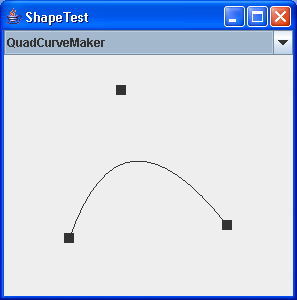# Polyline 2D : Shape « 2D Graphics GUI « Java

Polyline 2D

```

/*

Licensed to the Apache Software Foundation (ASF) under one or more
contributor license agreements.  See the NOTICE file distributed with
The ASF licenses this file to You under the Apache License, Version 2.0
(the "License"); you may not use this file except in compliance with

Unless required by applicable law or agreed to in writing, software
WITHOUT WARRANTIES OR CONDITIONS OF ANY KIND, either express or implied.
See the License for the specific language governing permissions and

*/

import java.awt.Point;
import java.awt.Polygon;
import java.awt.Rectangle;
import java.awt.Shape;
import java.awt.geom.AffineTransform;
import java.awt.geom.GeneralPath;
import java.awt.geom.Line2D;
import java.awt.geom.PathIterator;
import java.awt.geom.Point2D;
import java.awt.geom.Rectangle2D;
import java.io.Serializable;

/**
* This class has the same behavior than {@link Polygon2D}, except that
* the figure is not closed.
*
* @version \$Id: Polyline2D.java 594018 2007-11-12 04:17:41Z cam \$
*/
public class Polyline2D implements Shape, Cloneable, Serializable {

private static final float ASSUME_ZERO = 0.001f;

/**
* The total number of points.  The value of <code>npoints</code>
* represents the number of points in this <code>Polyline2D</code>.
*
*/
public int npoints;

/**
* The array of <i>x</i> coordinates. The value of {@link #npoints npoints} is equal to the
* number of points in this <code>Polyline2D</code>.
*
*/
public float[] xpoints;

/**
* The array of <i>x</i> coordinates. The value of {@link #npoints npoints} is equal to the
* number of points in this <code>Polyline2D</code>.
*
*/
public float[] ypoints;

/**
* Bounds of the Polyline2D.
* @see #getBounds()
*/
protected Rectangle2D bounds;

private GeneralPath path;
private GeneralPath closedPath;

/**
* Creates an empty Polyline2D.
*/
public Polyline2D() {
xpoints = new float;
ypoints = new float;
}

/**
* Constructs and initializes a <code>Polyline2D</code> from the specified
* parameters.
* @param xpoints an array of <i>x</i> coordinates
* @param ypoints an array of <i>y</i> coordinates
* @param npoints the total number of points in the
*                                <code>Polyline2D</code>
* @exception  NegativeArraySizeException if the value of
*                       <code>npoints</code> is negative.
* @exception  IndexOutOfBoundsException if <code>npoints</code> is
*             greater than the length of <code>xpoints</code>
*             or the length of <code>ypoints</code>.
* @exception  NullPointerException if <code>xpoints</code> or
*             <code>ypoints</code> is <code>null</code>.
*/
public Polyline2D(float[] xpoints, float[] ypoints, int npoints) {
if (npoints > xpoints.length || npoints > ypoints.length) {
throw new IndexOutOfBoundsException("npoints > xpoints.length || npoints > ypoints.length");
}
this.npoints = npoints;
this.xpoints = new float[npoints+1];   // make space for one more to close the polyline
this.ypoints = new float[npoints+1];   // make space for one more to close the polyline
System.arraycopy(xpoints, 0, this.xpoints, 0, npoints);
System.arraycopy(ypoints, 0, this.ypoints, 0, npoints);
calculatePath();
}

/**
* Constructs and initializes a <code>Polyline2D</code> from the specified
* parameters.
* @param xpoints an array of <i>x</i> coordinates
* @param ypoints an array of <i>y</i> coordinates
* @param npoints the total number of points in the <code>Polyline2D</code>
* @exception  NegativeArraySizeException if the value of
*                       <code>npoints</code> is negative.
* @exception  IndexOutOfBoundsException if <code>npoints</code> is
*             greater than the length of <code>xpoints</code>
*             or the length of <code>ypoints</code>.
* @exception  NullPointerException if <code>xpoints</code> or
*             <code>ypoints</code> is <code>null</code>.
*/
public Polyline2D(int[] xpoints, int[] ypoints, int npoints) {
if (npoints > xpoints.length || npoints > ypoints.length) {
throw new IndexOutOfBoundsException("npoints > xpoints.length || npoints > ypoints.length");
}
this.npoints = npoints;
this.xpoints = new float[npoints];
this.ypoints = new float[npoints];
for (int i = 0; i < npoints; i++) {
this.xpoints[i] = xpoints[i];
this.ypoints[i] = ypoints[i];
}
calculatePath();
}

public Polyline2D(Line2D line) {
npoints = 2;
xpoints = new float;
ypoints = new float;
xpoints = (float)line.getX1();
xpoints = (float)line.getX2();
ypoints = (float)line.getY1();
ypoints = (float)line.getY2();
calculatePath();
}

/**
* Resets this <code>Polyline2D</code> object to an empty polygon.
* The coordinate arrays and the data in them are left untouched
* but the number of points is reset to zero to mark the old
* vertex data as invalid and to start accumulating new vertex
* data at the beginning.
* All internally-cached data relating to the old vertices
* Note that since the coordinate arrays from before the reset
* are reused, creating a new empty <code>Polyline2D</code> might
* be more memory efficient than resetting the current one if
* the number of vertices in the new polyline data is significantly
* smaller than the number of vertices in the data from before the
* reset.
*/
public void reset() {
npoints = 0;
bounds = null;
path = new GeneralPath();
closedPath = null;
}

public Object clone() {
Polyline2D pol = new Polyline2D();
for (int i = 0; i < npoints; i++) {
}
return pol;
}

private void calculatePath() {
path = new GeneralPath();
path.moveTo(xpoints, ypoints);
for (int i = 1; i < npoints; i++) {
path.lineTo(xpoints[i], ypoints[i]);
}
bounds = path.getBounds2D();
closedPath = null;
}

private void updatePath(float x, float y) {
closedPath = null;
if (path == null) {
path = new GeneralPath(GeneralPath.WIND_EVEN_ODD);
path.moveTo(x, y);
bounds = new Rectangle2D.Float(x, y, 0, 0);
} else {
path.lineTo(x, y);
float _xmax = (float)bounds.getMaxX();
float _ymax = (float)bounds.getMaxY();
float _xmin = (float)bounds.getMinX();
float _ymin = (float)bounds.getMinY();
if (x < _xmin) _xmin = x;
else if (x > _xmax) _xmax = x;
if (y < _ymin) _ymin = y;
else if (y > _ymax) _ymax = y;
bounds = new Rectangle2D.Float(_xmin, _ymin, _xmax - _xmin, _ymax - _ymin);
}
}

}

/**
* Appends the specified coordinates to this <code>Polyline2D</code>.
* <p>
* If an operation that calculates the bounding box of this
* <code>Polyline2D</code> has already been performed, such as
* <code>getBounds</code> or <code>contains</code>, then this
* method updates the bounding box.
* @param       x the specified x coordinate
* @param       y the specified y coordinate
* @see         java.awt.Polygon#getBounds
* @see         java.awt.Polygon#contains(double,double)
*/
public void addPoint(float x, float y) {
if (npoints == xpoints.length) {
float[] tmp;

tmp = new float[npoints * 2];
System.arraycopy(xpoints, 0, tmp, 0, npoints);
xpoints = tmp;

tmp = new float[npoints * 2];
System.arraycopy(ypoints, 0, tmp, 0, npoints);
ypoints = tmp;
}
xpoints[npoints] = x;
ypoints[npoints] = y;
npoints++;
updatePath(x, y);
}

/**
* Gets the bounding box of this <code>Polyline2D</code>.
* The bounding box is the smallest {@link Rectangle} whose
* sides are parallel to the x and y axes of the
* coordinate space, and can completely contain the <code>Polyline2D</code>.
* @return a <code>Rectangle</code> that defines the bounds of this
* <code>Polyline2D</code>.
*/
public Rectangle getBounds() {
if (bounds == null) return null;
else return bounds.getBounds();
}

private void updateComputingPath() {
if (npoints >= 1) {
if (closedPath == null) {
closedPath = (GeneralPath)path.clone();
closedPath.closePath();
}
}
}

/**
* Determines whether the specified {@link Point} is inside this
* <code>Polyline2D</code>.
* This method is required to implement the Shape interface,
* but in the case of Line2D objects it always returns false since a line contains no area.
*/
public boolean contains(Point p) {
return false;
}

/**
* Determines if the specified coordinates are inside this
* <code>Polyline2D</code>.
* This method is required to implement the Shape interface,
* but in the case of Line2D objects it always returns false since a line contains no area.
*/
public boolean contains(double x, double y) {
return false;
}

/**
* Determines whether the specified coordinates are inside this
* <code>Polyline2D</code>.
* This method is required to implement the Shape interface,
* but in the case of Line2D objects it always returns false since a line contains no area.
*/
public boolean contains(int x, int y) {
return false;
}

/**
* Returns the high precision bounding box of the {@link Shape}.
* @return a {@link Rectangle2D} that precisely
*                bounds the <code>Shape</code>.
*/
public Rectangle2D getBounds2D() {
return bounds;
}

/**
* Tests if a specified {@link Point2D} is inside the boundary of this
* <code>Polyline2D</code>.
* This method is required to implement the Shape interface,
* but in the case of Line2D objects it always returns false since a line contains no area.
*/
public boolean contains(Point2D p) {
return false;
}

/**
* Tests if the interior of this <code>Polygon</code> intersects the
* interior of a specified set of rectangular coordinates.
* @param x the x coordinate of the specified rectangular
*                        shape's top-left corner
* @param y the y coordinate of the specified rectangular
*                        shape's top-left corner
* @param w the width of the specified rectangular shape
* @param h the height of the specified rectangular shape
* @return <code>true</code> if the interior of this
*                        <code>Polygon</code> and the interior of the
*                        specified set of rectangular
*                         coordinates intersect each other;
*                        <code>false</code> otherwise.
*/
public boolean intersects(double x, double y, double w, double h) {
if (npoints <= 0 || !bounds.intersects(x, y, w, h)) {
return false;
}
updateComputingPath();
return closedPath.intersects(x, y, w, h);
}

/**
* Tests if the interior of this <code>Polygon</code> intersects the
* interior of a specified <code>Rectangle2D</code>.
* @param r a specified <code>Rectangle2D</code>
* @return <code>true</code> if this <code>Polygon</code> and the
*                         interior of the specified <code>Rectangle2D</code>
*                         intersect each other; <code>false</code>
*                         otherwise.
*/
public boolean intersects(Rectangle2D r) {
return intersects(r.getX(), r.getY(), r.getWidth(), r.getHeight());
}

/**
* Tests if the interior of this <code>Polyline2D</code> entirely
* contains the specified set of rectangular coordinates.
* This method is required to implement the Shape interface,
* but in the case of Line2D objects it always returns false since a line contains no area.
*/
public boolean contains(double x, double y, double w, double h) {
return false;
}

/**
* Tests if the interior of this <code>Polyline2D</code> entirely
* contains the specified <code>Rectangle2D</code>.
* This method is required to implement the Shape interface,
* but in the case of Line2D objects it always returns false since a line contains no area.
*/
public boolean contains(Rectangle2D r) {
return false;
}

/**
* Returns an iterator object that iterates along the boundary of this
* of the outline of this <code>Polygon</code>.  An optional
* {@link AffineTransform} can be specified so that the coordinates
* returned in the iteration are transformed accordingly.
* @param at an optional <code>AffineTransform</code> to be applied to the
*                 coordinates as they are returned in the iteration, or
*                <code>null</code> if untransformed coordinates are desired
*                geometry of this <code>Polygon</code>.
*/
public PathIterator getPathIterator(AffineTransform at) {
if (path == null) return null;
else return path.getPathIterator(at);
}

/* get the associated {@link Polygon2D}.
* This method take care that may be the last point can
* be equal to the first. In that case it must not be included in the Polygon,
* as polygons declare their first point only once.
*/
public Polygon2D getPolygon2D() {
Polygon2D pol = new Polygon2D();
for (int i = 0; i < npoints - 1; i++) {
}
Point2D.Double p0 =
new Point2D.Double(xpoints, ypoints);
Point2D.Double p1 =
new Point2D.Double(xpoints[npoints-1], ypoints[npoints-1]);

if (p0.distance(p1) > ASSUME_ZERO)

return pol;
}

/**
* Returns an iterator object that iterates along the boundary of
* outline of the <code>Shape</code>.  Only SEG_MOVETO and SEG_LINETO, point types
* are returned by the iterator.
* Since polylines are already flat, the <code>flatness</code> parameter
* is ignored.
* @param at an optional <code>AffineTransform</code> to be applied to the
*                 coordinates as they are returned in the iteration, or
*                <code>null</code> if untransformed coordinates are desired
* @param flatness the maximum amount that the control points
*                 for a given curve can vary from colinear before a subdivided
*                curve is replaced by a straight line connecting the
*                 endpoints.  Since polygons are already flat the
*                 <code>flatness</code> parameter is ignored.
*                 <code>Shape</code> object's geometry.
*/
public PathIterator getPathIterator(AffineTransform at, double flatness) {
return path.getPathIterator(at);
}
}

/*

Licensed to the Apache Software Foundation (ASF) under one or more
contributor license agreements.  See the NOTICE file distributed with
The ASF licenses this file to You under the Apache License, Version 2.0
(the "License"); you may not use this file except in compliance with

Unless required by applicable law or agreed to in writing, software
WITHOUT WARRANTIES OR CONDITIONS OF ANY KIND, either express or implied.
See the License for the specific language governing permissions and

*/

/**
* This class is a Polygon with float coordinates.
*
* @version \$Id: Polygon2D.java 594018 2007-11-12 04:17:41Z cam \$
*/
class Polygon2D implements Shape, Cloneable, Serializable {

/**
* The total number of points.  The value of <code>npoints</code>
* represents the number of valid points in this <code>Polygon</code>.
*
*/
public int npoints;

/**
* The array of <i>x</i> coordinates. The value of {@link #npoints npoints} is equal to the
* number of points in this <code>Polygon2D</code>.
*
*/
public float[] xpoints;

/**
* The array of <i>x</i> coordinates. The value of {@link #npoints npoints} is equal to the
* number of points in this <code>Polygon2D</code>.
*
*/
public float[] ypoints;

/**
* Bounds of the Polygon2D.
* @see #getBounds()
*/
protected Rectangle2D bounds;

private GeneralPath path;
private GeneralPath closedPath;

/**
* Creates an empty Polygon2D.
*/
public Polygon2D() {
xpoints = new float;
ypoints = new float;
}

/**
* Constructs and initializes a <code>Polygon2D</code> from the specified
* Rectangle2D.
* @param rec the Rectangle2D
* @exception  NullPointerException rec is <code>null</code>.
*/
public Polygon2D(Rectangle2D rec) {
if (rec == null) {
throw new IndexOutOfBoundsException("null Rectangle");
}
npoints = 4;
xpoints = new float;
ypoints = new float;
xpoints = (float)rec.getMinX();
ypoints = (float)rec.getMinY();
xpoints = (float)rec.getMaxX();
ypoints = (float)rec.getMinY();
xpoints = (float)rec.getMaxX();
ypoints = (float)rec.getMaxY();
xpoints = (float)rec.getMinX();
ypoints = (float)rec.getMaxY();
calculatePath();
}

/**
* Constructs and initializes a <code>Polygon2D</code> from the specified
* Polygon.
* @param pol the Polygon
* @exception  NullPointerException pol is <code>null</code>.
*/
public Polygon2D(Polygon pol) {
if (pol == null) {
throw new IndexOutOfBoundsException("null Polygon");
}
this.npoints = pol.npoints;
this.xpoints = new float[pol.npoints];
this.ypoints = new float[pol.npoints];
for (int i = 0; i < pol.npoints; i++) {
xpoints[i] = pol.xpoints[i];
ypoints[i] = pol.ypoints[i];
}
calculatePath();
}

/**
* Constructs and initializes a <code>Polygon2D</code> from the specified
* parameters.
* @param xpoints an array of <i>x</i> coordinates
* @param ypoints an array of <i>y</i> coordinates
* @param npoints the total number of points in the <code>Polygon2D</code>
* @exception  NegativeArraySizeException if the value of
*                       <code>npoints</code> is negative.
* @exception  IndexOutOfBoundsException if <code>npoints</code> is
*             greater than the length of <code>xpoints</code>
*             or the length of <code>ypoints</code>.
* @exception  NullPointerException if <code>xpoints</code> or
*             <code>ypoints</code> is <code>null</code>.
*/
public Polygon2D(float[] xpoints, float[] ypoints, int npoints) {
if (npoints > xpoints.length || npoints > ypoints.length) {
throw new IndexOutOfBoundsException("npoints > xpoints.length || npoints > ypoints.length");
}
this.npoints = npoints;
this.xpoints = new float[npoints];
this.ypoints = new float[npoints];
System.arraycopy(xpoints, 0, this.xpoints, 0, npoints);
System.arraycopy(ypoints, 0, this.ypoints, 0, npoints);
calculatePath();
}

/**
* Constructs and initializes a <code>Polygon2D</code> from the specified
* parameters.
* @param xpoints an array of <i>x</i> coordinates
* @param ypoints an array of <i>y</i> coordinates
* @param npoints the total number of points in the <code>Polygon2D</code>
* @exception  NegativeArraySizeException if the value of
*                       <code>npoints</code> is negative.
* @exception  IndexOutOfBoundsException if <code>npoints</code> is
*             greater than the length of <code>xpoints</code>
*             or the length of <code>ypoints</code>.
* @exception  NullPointerException if <code>xpoints</code> or
*             <code>ypoints</code> is <code>null</code>.
*/
public Polygon2D(int[] xpoints, int[] ypoints, int npoints) {
if (npoints > xpoints.length || npoints > ypoints.length) {
throw new IndexOutOfBoundsException("npoints > xpoints.length || npoints > ypoints.length");
}
this.npoints = npoints;
this.xpoints = new float[npoints];
this.ypoints = new float[npoints];
for (int i = 0; i < npoints; i++) {
this.xpoints[i] = xpoints[i];
this.ypoints[i] = ypoints[i];
}
calculatePath();
}

/**
* Resets this <code>Polygon</code> object to an empty polygon.
*/
public void reset() {
npoints = 0;
bounds = null;
path = new GeneralPath();
closedPath = null;
}

public Object clone() {
Polygon2D pol = new Polygon2D();
for (int i = 0; i < npoints; i++) {
}
return pol;
}

private void calculatePath() {
path = new GeneralPath();
path.moveTo(xpoints, ypoints);
for (int i = 1; i < npoints; i++) {
path.lineTo(xpoints[i], ypoints[i]);
}
bounds = path.getBounds2D();
closedPath = null;
}

private void updatePath(float x, float y) {
closedPath = null;
if (path == null) {
path = new GeneralPath(GeneralPath.WIND_EVEN_ODD);
path.moveTo(x, y);
bounds = new Rectangle2D.Float(x, y, 0, 0);
} else {
path.lineTo(x, y);
float _xmax = (float)bounds.getMaxX();
float _ymax = (float)bounds.getMaxY();
float _xmin = (float)bounds.getMinX();
float _ymin = (float)bounds.getMinY();
if (x < _xmin) _xmin = x;
else if (x > _xmax) _xmax = x;
if (y < _ymin) _ymin = y;
else if (y > _ymax) _ymax = y;
bounds = new Rectangle2D.Float(_xmin, _ymin, _xmax - _xmin, _ymax - _ymin);
}
}

/* get the associated {@link Polyline2D}.
*/
public Polyline2D getPolyline2D() {

Polyline2D pol = new Polyline2D( xpoints, ypoints, npoints );

return pol;
}

public Polygon getPolygon() {
int[] _xpoints = new int[npoints];
int[] _ypoints = new int[npoints];
for (int i = 0; i < npoints; i++) {
_xpoints[i] = (int)xpoints[i];     // todo maybe rounding is better ?
_ypoints[i] = (int)ypoints[i];
}

return new Polygon(_xpoints, _ypoints, npoints);
}

}

/**
* Appends the specified coordinates to this <code>Polygon2D</code>.
* @param       x the specified x coordinate
* @param       y the specified y coordinate
*/
public void addPoint(float x, float y) {
if (npoints == xpoints.length) {
float[] tmp;

tmp = new float[npoints * 2];
System.arraycopy(xpoints, 0, tmp, 0, npoints);
xpoints = tmp;

tmp = new float[npoints * 2];
System.arraycopy(ypoints, 0, tmp, 0, npoints);
ypoints = tmp;
}
xpoints[npoints] = x;
ypoints[npoints] = y;
npoints++;
updatePath(x, y);
}

/**
* Determines whether the specified {@link Point} is inside this
* <code>Polygon</code>.
* @param p the specified <code>Point</code> to be tested
* @return <code>true</code> if the <code>Polygon</code> contains the
*                         <code>Point</code>; <code>false</code> otherwise.
* @see #contains(double, double)
*/
public boolean contains(Point p) {
return contains(p.x, p.y);
}

/**
* Determines whether the specified coordinates are inside this
* <code>Polygon</code>.
* <p>
* @param x the specified x coordinate to be tested
* @param y the specified y coordinate to be tested
* @return  <code>true</code> if this <code>Polygon</code> contains
*                         the specified coordinates, (<i>x</i>,&nbsp;<i>y</i>);
*                         <code>false</code> otherwise.
*/
public boolean contains(int x, int y) {
return contains((double) x, (double) y);
}

/**
* Returns the high precision bounding box of the {@link Shape}.
* @return a {@link Rectangle2D} that precisely
*                bounds the <code>Shape</code>.
*/
public Rectangle2D getBounds2D() {
return bounds;
}

public Rectangle getBounds() {
if (bounds == null) return null;
else return bounds.getBounds();
}

/**
* Determines if the specified coordinates are inside this
* <code>Polygon</code>.  For the definition of
* @param x the specified x coordinate
* @param y the specified y coordinate
* @return <code>true</code> if the <code>Polygon</code> contains the
* specified coordinates; <code>false</code> otherwise.
*/
public boolean contains(double x, double y) {
if (npoints <= 2 || !bounds.contains(x, y)) {
return false;
}
updateComputingPath();

return closedPath.contains(x, y);
}

private void updateComputingPath() {
if (npoints >= 1) {
if (closedPath == null) {
closedPath = (GeneralPath)path.clone();
closedPath.closePath();
}
}
}

/**
* Tests if a specified {@link Point2D} is inside the boundary of this
* <code>Polygon</code>.
* @param p a specified <code>Point2D</code>
* @return <code>true</code> if this <code>Polygon</code> contains the
*                 specified <code>Point2D</code>; <code>false</code>
*          otherwise.
* @see #contains(double, double)
*/
public boolean contains(Point2D p) {
return contains(p.getX(), p.getY());
}

/**
* Tests if the interior of this <code>Polygon</code> intersects the
* interior of a specified set of rectangular coordinates.
* @param x the x coordinate of the specified rectangular
*                        shape's top-left corner
* @param y the y coordinate of the specified rectangular
*                        shape's top-left corner
* @param w the width of the specified rectangular shape
* @param h the height of the specified rectangular shape
* @return <code>true</code> if the interior of this
*                        <code>Polygon</code> and the interior of the
*                        specified set of rectangular
*                         coordinates intersect each other;
*                        <code>false</code> otherwise.
*/
public boolean intersects(double x, double y, double w, double h) {
if (npoints <= 0 || !bounds.intersects(x, y, w, h)) {
return false;
}
updateComputingPath();
return closedPath.intersects(x, y, w, h);
}

/**
* Tests if the interior of this <code>Polygon</code> intersects the
* interior of a specified <code>Rectangle2D</code>.
* @param r a specified <code>Rectangle2D</code>
* @return <code>true</code> if this <code>Polygon</code> and the
*                         interior of the specified <code>Rectangle2D</code>
*                         intersect each other; <code>false</code>
*                         otherwise.
*/
public boolean intersects(Rectangle2D r) {
return intersects(r.getX(), r.getY(), r.getWidth(), r.getHeight());
}

/**
* Tests if the interior of this <code>Polygon</code> entirely
* contains the specified set of rectangular coordinates.
* @param x the x coordinate of the top-left corner of the
*                         specified set of rectangular coordinates
* @param y the y coordinate of the top-left corner of the
*                         specified set of rectangular coordinates
* @param w the width of the set of rectangular coordinates
* @param h the height of the set of rectangular coordinates
* @return <code>true</code> if this <code>Polygon</code> entirely
*                         contains the specified set of rectangular
*                         coordinates; <code>false</code> otherwise.
*/
public boolean contains(double x, double y, double w, double h) {
if (npoints <= 0 || !bounds.intersects(x, y, w, h)) {
return false;
}

updateComputingPath();
return closedPath.contains(x, y, w, h);
}

/**
* Tests if the interior of this <code>Polygon</code> entirely
* contains the specified <code>Rectangle2D</code>.
* @param r the specified <code>Rectangle2D</code>
* @return <code>true</code> if this <code>Polygon</code> entirely
*                         contains the specified <code>Rectangle2D</code>;
*                        <code>false</code> otherwise.
* @see #contains(double, double, double, double)
*/
public boolean contains(Rectangle2D r) {
return contains(r.getX(), r.getY(), r.getWidth(), r.getHeight());
}

/**
* Returns an iterator object that iterates along the boundary of this
* of the outline of this <code>Polygon</code>.  An optional
* {@link AffineTransform} can be specified so that the coordinates
* returned in the iteration are transformed accordingly.
* @param at an optional <code>AffineTransform</code> to be applied to the
*                 coordinates as they are returned in the iteration, or
*                <code>null</code> if untransformed coordinates are desired
*                geometry of this <code>Polygon</code>.
*/
public PathIterator getPathIterator(AffineTransform at) {
updateComputingPath();
if (closedPath == null) return null;
else return closedPath.getPathIterator(at);
}

/**
* Returns an iterator object that iterates along the boundary of
* outline of the <code>Shape</code>.  Only SEG_MOVETO, SEG_LINETO, and
* SEG_CLOSE point types are returned by the iterator.
* Since polygons are already flat, the <code>flatness</code> parameter
* is ignored.
* @param at an optional <code>AffineTransform</code> to be applied to the
*                 coordinates as they are returned in the iteration, or
*                <code>null</code> if untransformed coordinates are desired
* @param flatness the maximum amount that the control points
*                 for a given curve can vary from colinear before a subdivided
*                curve is replaced by a straight line connecting the
*                 endpoints.  Since polygons are already flat the
*                 <code>flatness</code> parameter is ignored.
*                 <code>Shape</code> object's geometry.
*/
public PathIterator getPathIterator(AffineTransform at, double flatness) {
return getPathIterator(at);
}
}

```

### Related examples in the same category

 1 Creating Basic Shapes 2 fillRect (int, int, int, int) method draws a solid rectangle 3 Creating a Shape Using Lines and Curves 4 Combining Shapes 5 Draw rectangles, use the drawRect() method. To fill rectangles, use the fillRect() method 6 Draw line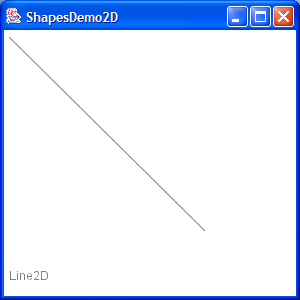7 Draw a Polygon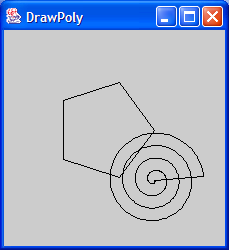8 Draw an oval outline 9 Draw a (Round)rectangle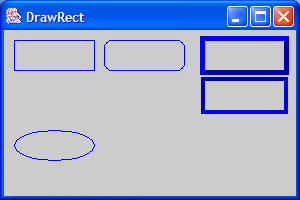10 Fill a polygon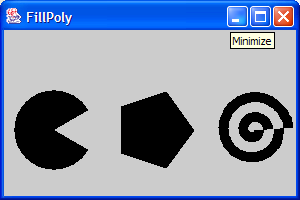11 Fill a solid oval 12 Fill a (Round)rectangle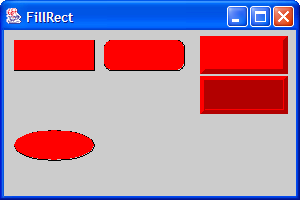13 Change font14 Draw rectangle 2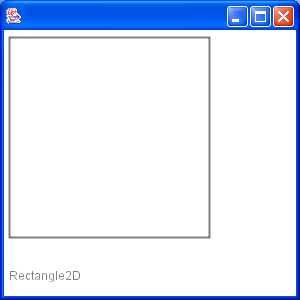15 Draw Arc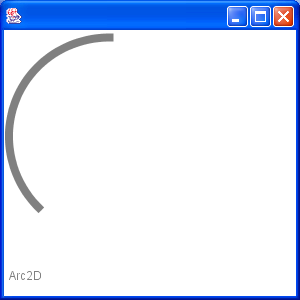16 Draw Ellipse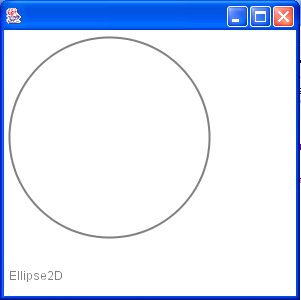17 Fill a Rectangle 2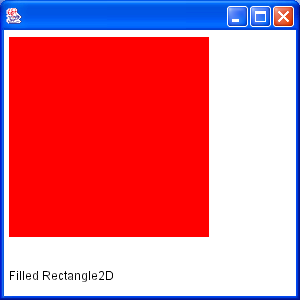18 Fill Arc 2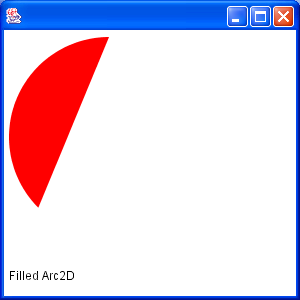19 Draw text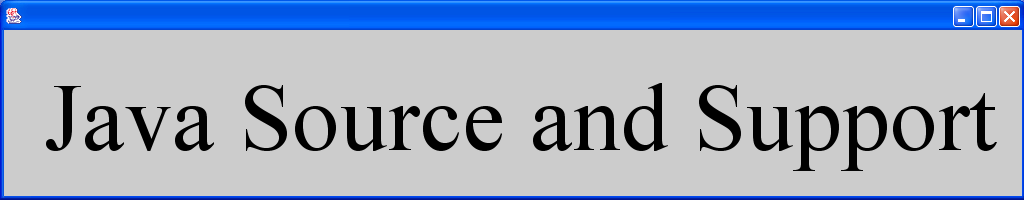20 Draw unicode string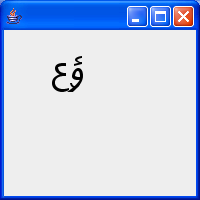21 Shape combine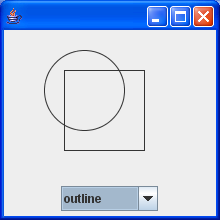22 Effects23 Mouse drag and drop to draw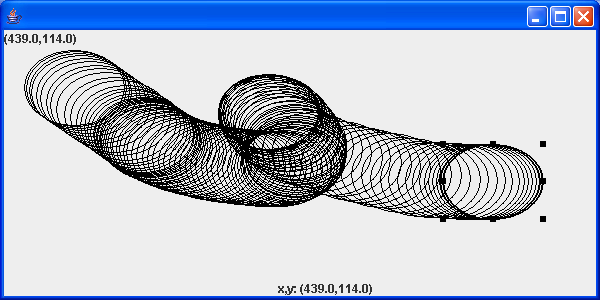24 Arc demonstration: scale, move, rotate, sheer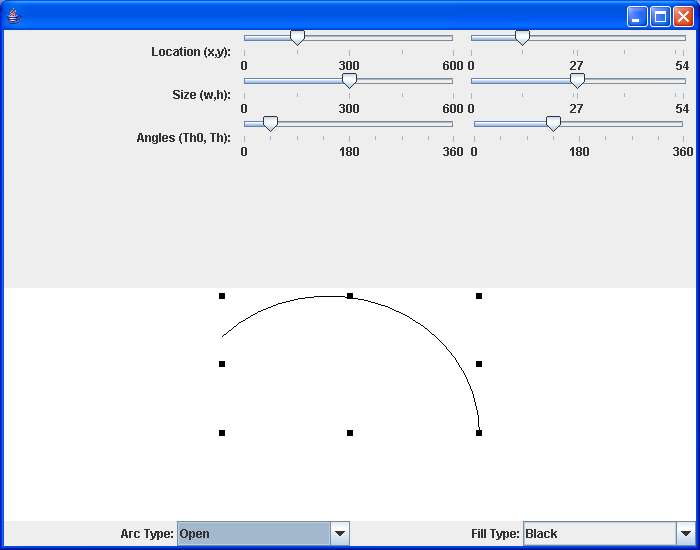25 Hypnosis Spiral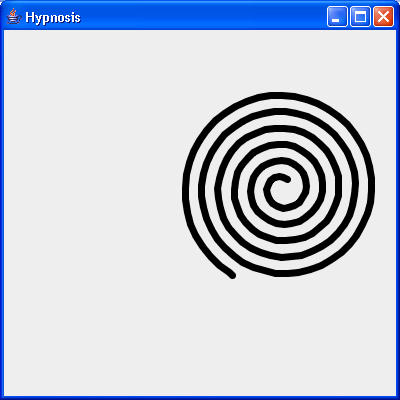26 GlyphVector.getNumGlyphs() 27 Resize a shape 28 Rectangle with rounded corners drawn using Java 2D Graphics API 29 Compares two ellipses and returns true if they are equal or both null. 30 Compares two lines are returns true if they are equal or both null. 31 Creates a diagonal cross shape. 32 Creates a diamond shape. 33 Creates a new Stroke-Object for the given type and with. 34 Creates a region surrounding a line segment by 'widening' the line segment. 35 Creates a triangle shape that points downwards. 36 Creates a triangle shape that points upwards. 37 Generate Polygon 38 Polygon with float coordinates. 39 Serialises a Shape object. 40 Tests two polygons for equality. If both are null this method returns true. 41 Union two rectangles 42 Calculate Intersection Clip 43 Draws a shape with the specified rotation about (x, y). 44 Checks, whether the given rectangle1 fully contains rectangle 2 (even if rectangle 2 has a height or width of zero!). 45 Reads a Point2D object that has been serialised by the writePoint2D(Point2D, ObjectOutputStream)} method. 46 Returns a point based on (x, y) but constrained to be within the bounds of a given rectangle. 47 RectListManager is a class to manage a list of rectangular regions. 48 Fill Rectangle2D.Double and Ellipse2D.Double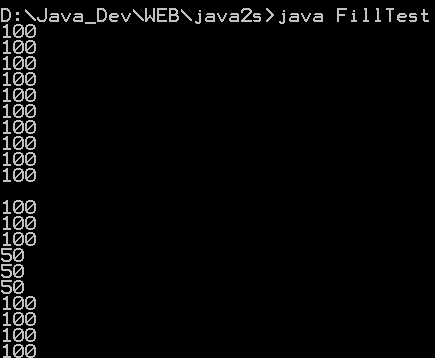49 This program demonstrates the various 2D shapes